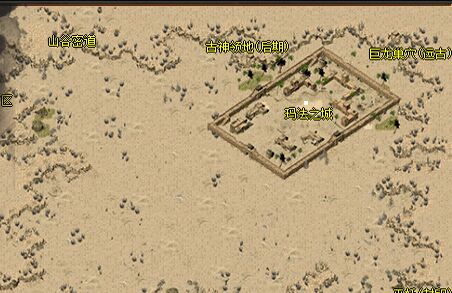# 七星辅助过天魔旗下天影沉默赤月峡谷脱机脚本如果你目前没有好服玩可以去体验一下，配合定制版传奇辅助来挂机，可以让你节省很多时间和金钱。让你在打金服中也能玩的嗨皮。持续关注七星辅助官网获取更多关于辅助的消息，

天魔旗下天影沉默算是一个比较好玩的版本，玩家在玩耍的时候还可以打金，如果只是自己手动打效率就很低。之前给传奇玩家介绍过全新的内存脱机挂，可以在这个服里自由的开启，现在七星辅助官网给大家来分享一个脱机脚本。

七星辅助官网分享最新定制挂过天魔旗下天影沉默赤月峡谷脱机脚本如下：

function 回收()
组件封包(3,271)
等待(1000)
选择("一键回收勾选的装备")
等待(1000)
组件封包(0,90)                            --刷新背包
end

local 地图名称 = 取地图名称()
if 地图名称 == "玛法之城" then
if 移动到坐标范围(78,30,0) == 1 then

end
elseif 地图名称 == "山谷密道" then
if 移动到坐标范围(45,23,0) == 1 then

end
elseif 地图名称 == "赤月峡谷" then
死亡小退()
if 取背包剩余空间() <= 2 then
回收()
else
执行打怪()
end
elseif 地图名称 == "天影大陆" then          --主城地图名
if 移动到坐标范围(117,119,5) == 1 then
if 点击NPC坐标(114,119) == 1 then
等待(700)
end

85%的户主都看了以下辅助挂机脚本下载